Open in App
Not now

# Excel Cell References

• Last Updated : 20 May, 2021

A cell reference, also known as a cell address, is a mechanism that defines a cell on a worksheet by combining a column letter and a row number. We can refer to any cell (in Excel formulas) in the worksheet by using the cell references.

For example :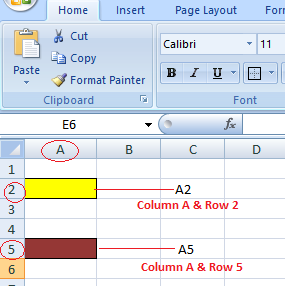Here we refer to the cell in column A & row 2 by A2 & cell in column A & row 5 by A5. You can make use of such notations in any of the formula or to copy the value of one cell to other cell (by using = A2 or = A5).

### Relative Cell Reference

A cell reference is by default a relative reference, which means that the reference is relative to the cell’s position.  If you refer to cell B1 from cell E1, for example, actually you would be referring to a cell that is 3 columns to the left (E-B = 3) within the same row number 1.

When it is copied to other locations present in a worksheet, the relative reference for that location will be changed automatically. (because relative cell reference describes offset to another cell rather than a fixed address as In our example offset is : 3 columns left in the same row).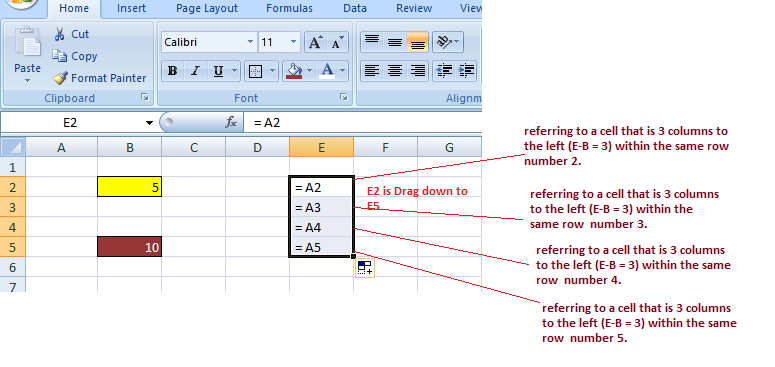Example 2: If you copy the formula = C2 / A2  from the cell “E2” to “E3”, the formula in E3 will automatically become =C3/A3.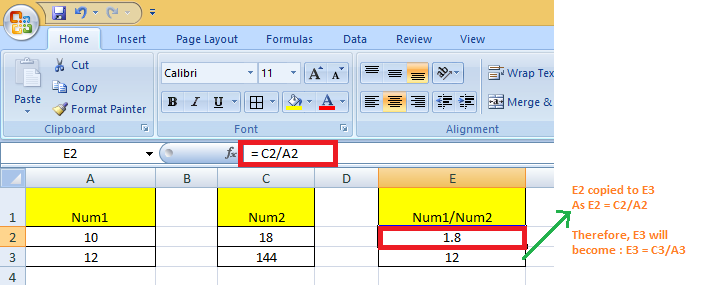### Absolute Cell Reference :

When copying or using AutoFill, there are times when the cell reference must stay the same. A column and/or row reference is kept constant using dollar signs. So, to get an absolute reference from a relative, we can use the dollar sign (\$) characters.

To refer to an actual fixed location on a worksheet whenever copying is done, we use absolute reference. The reference here is locked such that rows and columns do not shift when copied.

Here Dollar (\$) before row fixes the row & before the column fixes the column.

Example: When we fix both row & column – Say if we want to lock  row 2 & column A, we will use \$A\$2 as:

`G2 = C2/\$A\$2, when copied to G3, G3 becomes = C3/\$A\$2`

Note: C3 is 4 columns left to G3 in the same row.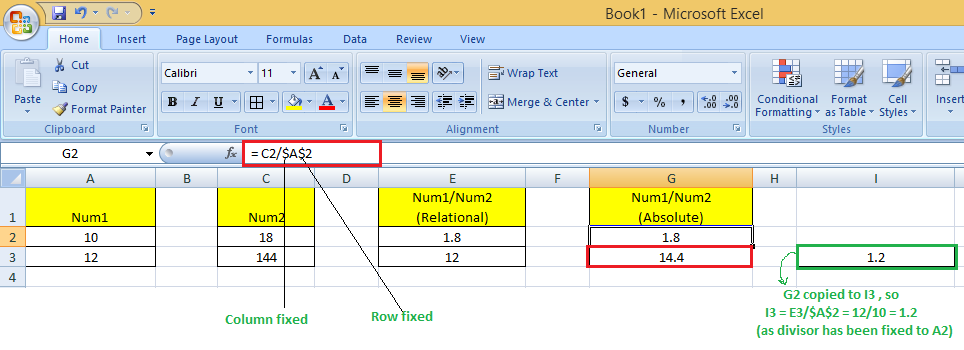Here original cell reference A2 is maintained whenever we copy G2 to any of the cells. So I3 = E3/\$A\$2 because E3 comes from the relative reference ( 4 columns left to the current one) & /\$A\$2  comes from the absolute reference.

Therefore, I3  = E3//\$A\$2 = 12/10 = 1.2

### Relative and absolute cell references for calculating dates

We can use relative and absolute cell references to calculate dates.

Example : To Calculate the Date of Delivery online from the given date of the order placed & no of days it will take to deliver :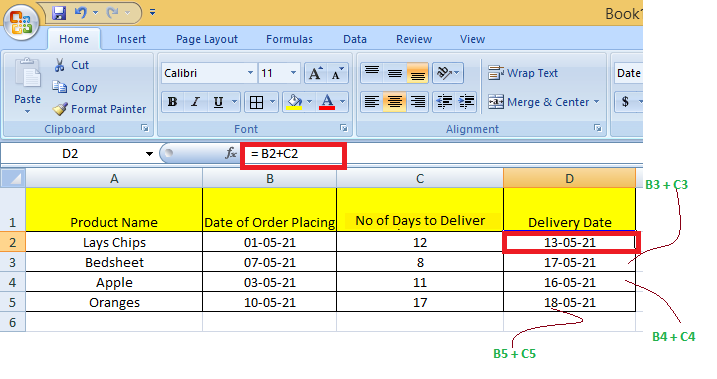Here, We calculate the Date of Delivery by = Order Date + No of days to deliver. We used Relative cell reference so that individual product delivery dates can be calculated.

Absolute cell references for calculating dates :

Example: To Calculate the Date of Birth When the age is known is a number of days using Current date can be done by making use of absolute reference.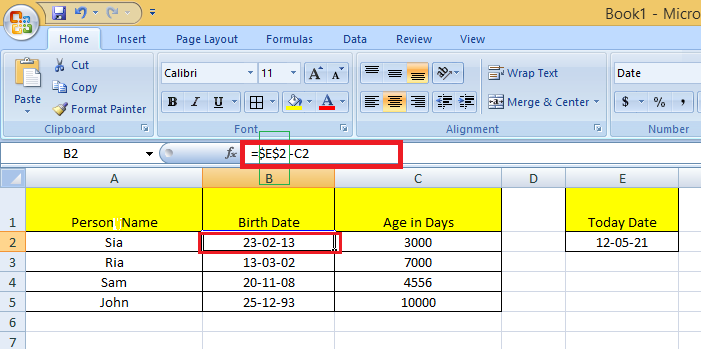Here, We calculate DOB by = Current Date – Age in days. The Current date is contained in the cell E2 & in subtraction, we fixed that date to subtract the days from.

### Excel Mixed Cell Reference

An absolute column and relative row, or an absolute row and relative column, is a mixed cell reference. You get an absolute column or absolute row when you individually put the \$ before the column letter or before the row number. Example : \$B8 is relative for row 8 but absolute for column B; and B\$8 is absolute for row 1 but relative for column A.

Here Dollar (\$) before row number fixes/locks the row & before the column name fixes/locks the column.

Example: When we fix the only row: If we have G2 = C2/A\$2 then :

We used \$ before the row number, so we are locking the only row here. When  G2 is copied to G3, G3 = C3/A\$2 (not C3/A3) because the row has been fixed already.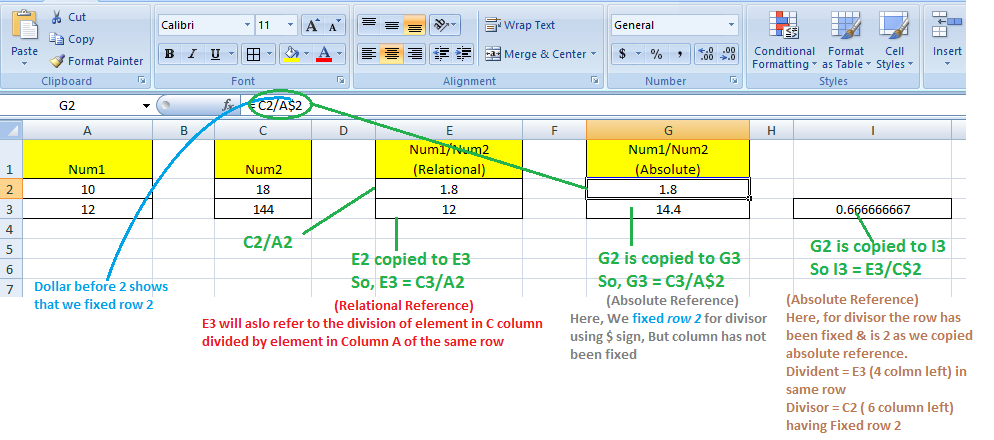Here, whenever we copy G2 to any other cell, always the divisor will refer to a fixed row 2 (column vary according to the concept of relative reference)

So, when G2 is copied to I3, I3 = E3/C\$2 because E3 comes from the relative reference (4 columns left to the current one) & C\$2  comes from the absolute reference for row & relative  reference for Column ( 6 Columns left to the current one)

### Whole Column Reference

You will want to refer to all the cells inside a particular column when operating with an Excel worksheet with any number of rows. Simply type a column letter twice with a colon in between to refer to the entire column B, for example, B:B.

Example: You may want to find the sum of a column of data in certain cases. While you can do this with a regular cell range, such as =SUM(B1:B10), you will need to change the cell range if your spreadsheet grows in size.

Excel, on the other hand, has a cell range that do not require the row number and takes all the cells in the column in action. If you wanted to find the sum of all the values in column B, for example, you would type =SUM (B:B). You can add as much data as you want to your spreadsheet without having to change your cell ranges if you use this type of cell range.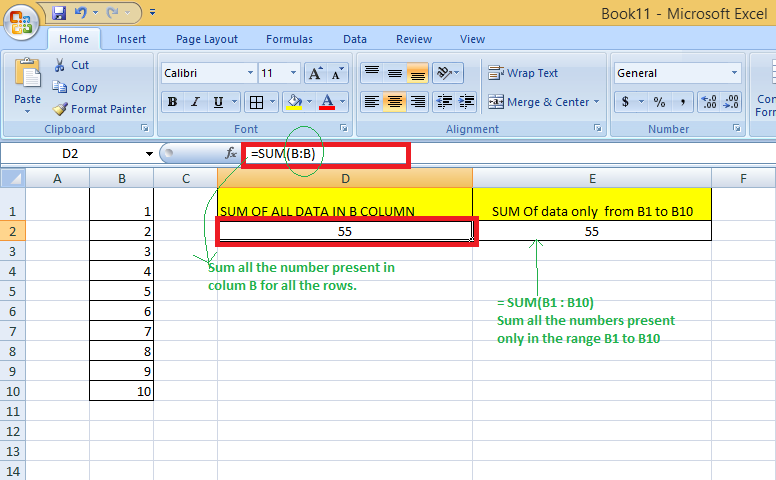### Whole Row Reference

You will want to refer to all the cells inside a particular row when operating with an Excel worksheet with any number of columns. Simply type a row number twice with a colon in between to refer to the entire row, for example, 2:2.

Example: You may want to find the sum of a row of data in certain cases. While you can do this with a regular cell range, such as =SUM(A2 : J2), you will need to change the cell range if your spreadsheet grows in size.

Excel, on the other hand, has a cell range that do not require the column letter and takes all the cells in the row in action. If you wanted to find the sum of all the values in row 2, for example, you would type =SUM (2:2). You can add as much data as you want to your spreadsheet without having to change your cell ranges if you use this type of cell range.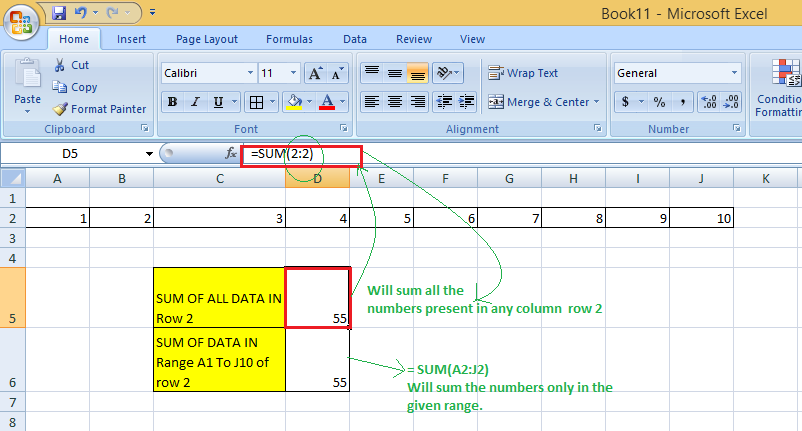### Refer to an Entire Column excluding the first few rows :

To refer to the entire column excluding the first few rows, you need to specify the range as we give in a normal fashion. We know that the Excel worksheets can have only 1,048,576 rows. (To check this go to an empty cell & press: Ctrl + Down arrow Key)

So, we can do the sum of the entire column B except for the first 5 rows by = SUM(B6:B1048576).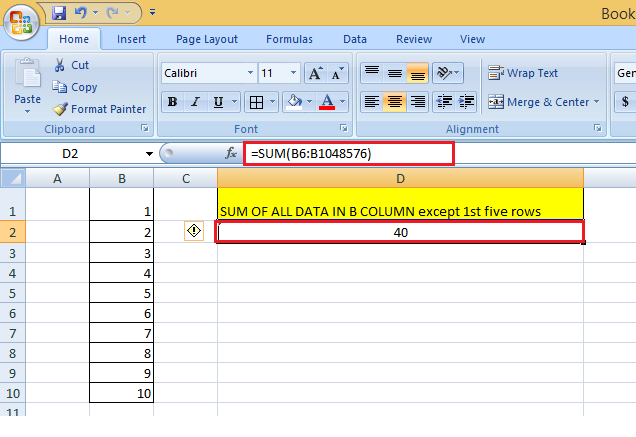### Using a Mixed Entire Column Reference in Excel

You can also create a mixed entire-column reference, say for example \$B:B. But, practically it is difficult to find a situation where it would be used.

Example :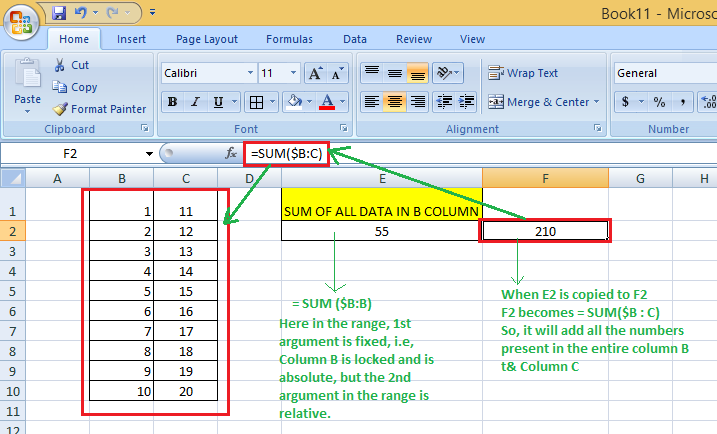### How to switch between Absolute, Relative, and Mixed References?

The \$ sign can be manually typed in an Excel formula to adjust a relative cell relation to absolute or mixed. You can also speed things up by pressing the F4 key. You must be in formula edit mode to use the F4 shortcut. The steps are :
Firstly, Choose the cell that contains the formula. Then by pressing the F2 key or double-clicking the cell, you can enter Edit mode. Select the cell reference in which you want to make changes. Then, switch between four-cell reference forms by pressing F4.

Example: When you select a cell having only relative reference (i.e., no \$ sign), say = B2:

• The first time when you press F4, it becomes =\$B\$2
• The second time when you press F4, it becomes =B\$2
• The third time when you press F4, it becomes=\$B2
• The fourth time when you press F4, it becomes back to the relative reference=B2
`B2 --Press F4--> =\$B\$2  --Press F4--> =B\$2 --Press F4--> = =\$B2  --Press F4--> =B2`

So, using F4, you do not require to manually type the \$ symbol.

My Personal Notes arrow_drop_up